Dealing with label switching: relabelling in Bayesian mixture models by pivotal units

2021-04-30

In this vignette we explore the fit of Gaussian mixture models and the relabelling pivotal method proposed in Egidi et al. (2018b) through the pivmet package. First of all, we load the package:

library(pivmet)
library(bayesmix)
library(bayesplot)

The pivmet package provides a simple framework to (i) fit univariate and multivariate mixture models according to a Bayesian flavour and detect the pivotal units, via the piv_MCMC function; (ii) perform the relabelling step via the piv_rel function.

There are two main functions used for this task.

The function piv_MCMC:

• performs MCMC sampling for Gaussian mixture models using the underlying rjags or rstan packages (chosen by the users through the optional function argument software, by default set to rjags). Precisely the package uses: the JAGSrun function of the bayesmix package for univariate mixture models; the run.jags function of the runjags package for bivariate mixture models; the stan function of the rstan package for both univariate and bivariate mixture models.

• Post-processes the chains and randomly switches their values (Frühwirth-Schnatter 2001).

• Builds a co-association matrix for the $$N$$ units. After $$H$$ MCMC iterations, the function implements a clustering procedure with $$k$$ groups, the user may choose among agglomerative or divisive hierarchical clustering through the optional argument clustering. Using the latent formulation for mixture models, we denote with $$[z_i]_h$$ the group allocation of the $$i$$-th unit at the $$h$$-th iteration. In such a way, a co-association matrix $$C$$ for the $$N$$ statistical units is built, where the single cell $$c_{ip}$$ is the fraction of times the unit $$i$$ and the unit $$p$$ belong to the same group along the $$H$$ MCMC iterations:

$c_{ip} = \frac{n_{ip}}{H}=\frac{1}{H} \sum_{h=1}^{H}|[z_i]_h=[z_p]_h|,$ where $$|\cdot|$$ denotes the event indicator and $$n_{ip}$$ is the number of times the units $$i, \ p$$ belong to the same group along the sampling.

• Extracts the pivots, one for each group, which are (pairwise) separated units with (posterior) probability one (that is, the posterior probability of any two of them being in the same group is approximately zero). We denote them by $$i_1,\ldots,i_k$$. The user may choose among four procedures for extracting the pivotal units with the optional argument piv.criterion. For group $$j$$ containing $$J_j$$ units, one can choose:

• $$i^{*}$$ that maximizes $$\sum_{p \in J_j}c_{ip}$$ ("maxsumint", default method);

• $$i^{*}$$ that maximizes $$\sum_{p \in J_j}c_{ip}- \sum_{p \not\in J_j}c_{ip}$$ ("maxsumdiff");

• $$i^{*}$$ that minimizes $$\sum_{p \not\in J_j}c_{ip}$$ ("minsumnoint").

These three methods are applied by the internal function piv_sel. Alternatively, when $$k <5$$, the user can set piv.criterion="MUS" (Egidi et al. 2018a) which performs a sequential search of identity submatrices within the matrix $$C$$ via the internal function MUS.

The function piv_rel:

• performs the relabelling algorithm using the $$k$$ pivotal units as groups identifiers. The pivotal units previously detected play a central role, yielding to the following relabelling for the $$h$$-th iteration:

\begin{align*} [\mu_{j}]_h=&[\mu_{z_{i_{j}}}]_h \\ [z_{i}]_h =j & \mbox{ for } i : [z_i]_h=[z_{i_{j}}]_h,\\ \end{align*} where $$\boldsymbol{\mu}=(\mu_1,\mu_2,\ldots,\mu_k)$$ is the vector of the means parameters and $$\boldsymbol{z}=(z_1,z_2,\ldots,z_N)$$ an i.i.d. vector of latent variables taking values in $$\{1,2,\ldots,k \}$$ and denoting the group membership of each statistical unit.

piv_rel takes as input the MCMC output from piv_MCMC and returns the relabelled chains and the corresponding posterior estimates.

Example: bivariate Gaussian data

Suppose now that $$\boldsymbol{y}_i \in \mathbb{R}^2$$ and assume that:

$\boldsymbol{y}_i \sim \sum_{j=1}^{k}\eta_{j}\mathcal{N}_{2}(\boldsymbol{\mu}_{j}, \boldsymbol{\Sigma})$ where $$\boldsymbol{\mu}_j$$ is the mean vector for group $$j$$, $$\boldsymbol{\Sigma}$$ is a positive-definite covariance matrix and the mixture weight $$\eta_j= P(z_i=j)$$ as for the one-dimensional case. We may generate Gaussian mixture data through the function piv_sim, specifying the sample size $$N$$, the desired number of groups $$k$$ and the $$k \times 2$$ matrix for the $$k$$ mean vectors. The argument W handles the weights for a nested mixture, in which the $$j$$-th component is in turn modelled as a two-component mixture, with covariance matrices $$\boldsymbol{\Sigma}_{p1}, \boldsymbol{\Sigma}_{p2}$$, respectively.

set.seed(500)
N  <- 200
k  <- 3
D <- 2
nMC <- 2000
M1 <- c(-10,8)
M2 <- c(10,.1)
M3 <- c(30,8)
# matrix of input means
Mu <- rbind(M1,M2,M3)
# covariance matrices for the two subgroups
Sigma.p1 <- diag(D)
Sigma.p2 <- (10^2)*diag(D)
# subgroups' weights
W   <- c(0.2,0.8)
# simulate data
sim <- piv_sim(N = N, k = k, Mu = Mu,
Sigma.p1 = Sigma.p1, Sigma.p2 = Sigma.p2, W = W)

The function piv_MCMC requires only three mandatory arguments: the data object y, the number of components k and the number of MCMC iterations, nMC. By default, it performs Gibbs sampling using the runjags package. If software="rjags", for bivariate data the priors are specified as:

\begin{align} \boldsymbol{\mu}_j \sim & \mathcal{N}_2(\boldsymbol{\mu}_0, S_2)\\ \Sigma^{-1} \sim & \mbox{Wishart}(S_3, 3)\\ \eta \sim & \mbox{Dirichlet}(\boldsymbol{\alpha}), \end{align}

where $$\boldsymbol{\alpha}$$ is a $$k$$-dimensional vector and $$S_2$$ and $$S_3$$ are positive definite matrices. By default, $$\boldsymbol{\mu}_0=\boldsymbol{0}$$, $$\boldsymbol{\alpha}=(1,\ldots,1)$$ and $$S_2$$ and $$S_3$$ are diagonal matrices, with diagonal elements equal to 1e+05. Different values can be specified for the hyperparameters $$\boldsymbol{\mu}_0, S_2, S_3$$ and $$\boldsymbol{\alpha}$$: priors =list(mu_0 = c(1,1), S2 = ..., S3 = ..., alpha = ...)}, with the constraint for $$S2$$ and $$S3$$ to be positive definite, and $$\boldsymbol{\alpha}$$ a vector of dimension $$k$$ with nonnegative elements.

If software="rstan", the function performs Hamiltonian Monte Carlo (HMC) sampling. In this case the priors are specified as:

\begin{align} \boldsymbol{\mu}_j \sim & \mathcal{N}_2(\boldsymbol{\mu}_0, LD^*L^{T})\\ L \sim & \mbox{LKJ}(\eta)\\ D_{1,2} \sim & \mbox{HalfCauchy}(0, \sigma_d). \end{align}

The covariance matrix is expressed in terms of the LDL decomposition as $$LD^*L^{T}$$, a variant of the classical Cholesky decomposition, where $$L$$ is a $$2 \times 2$$ lower unit triangular matrix and $$D^*$$ is a $$2 \times 2$$ diagonal matrix. The Cholesky correlation factor $$L$$ is assigned a LKJ prior with $$\epsilon$$ degrees of freedom, which, combined with priors on the standard deviations of each component, induces a prior on the covariance matrix; as $$\epsilon \rightarrow \infty$$ the magnitude of correlations between components decreases, whereas $$\epsilon=1$$ leads to a uniform prior distribution for $$L$$. By default, the hyperparameters are $$\boldsymbol{\mu}_0=\boldsymbol{0}$$, $$\sigma_d=2.5, \epsilon=1$$. Different values can be chosen with the argument: priors=list(mu_0=c(1,2), sigma_d = 4, epsilon = 2).

We fit the model using rjags with 2000 MCMC iterations and default priors:

res <- piv_MCMC(y = sim$y, k= k, nMC =nMC, piv.criterion = "maxsumdiff") #> Compiling rjags model... #> Calling the simulation using the rjags method... #> Note: the model did not require adaptation #> Burning in the model for 1000 iterations... #> Running the model for 2000 iterations... #> Simulation complete #> Note: Summary statistics were not produced as there are >50 monitored #> variables #> [To override this behaviour see ?add.summary and ?runjags.options] #> FALSEFinished running the simulation #> Calculating summary statistics... #> Calculating the Gelman-Rubin statistic for 13 variables.... #> #> JAGS model summary statistics from 8000 samples (chains = 4; adapt+burnin = 2000): #> #> Lower95 Median Upper95 Mean SD Mode MCerr #> mu[1,1] -14.829 -11.523 -8.0775 -11.327 49.623 -- 0.9133 #> mu[2,1] 5.4357 9.1102 12.601 8.7838 2.3082 -- 0.078065 #> mu[3,1] 27.54 31.294 34.6 31.112 1.8626 -- 0.051285 #> mu[1,2] 3.7495 6.9391 10.127 7.0995 51.981 -- 0.41223 #> mu[2,2] -1.9145 0.75295 3.3387 0.74767 1.3394 -- 0.026681 #> mu[3,2] 2.3062 5.4897 8.8554 5.4893 1.6887 -- 0.033315 #> tau[1,1] 0.010643 0.018154 0.025855 0.017913 0.0037111 -- 0.00011818 #> tau[2,1] -0.0027434 0.00063781 0.0043997 0.00068717 0.0018097 -- 0.000053598 #> tau[1,2] -0.0027434 0.00063781 0.0043997 0.00068717 0.0018097 -- 0.000053598 #> tau[2,2] 0.008687 0.010953 0.013372 0.011027 0.0012373 -- 0.000019645 #> eta 0.21841 0.32903 0.434 0.31988 0.071273 -- 0.0021263 #> eta 0.30784 0.42199 0.53787 0.42745 0.06351 -- 0.0015864 #> eta 0.17624 0.25015 0.32818 0.25267 0.039851 -- 0.00086819 #> #> MC%ofSD SSeff AC.10 psrf #> mu[1,1] 1.8 2952 -0.0081421 1.2905 #> mu[2,1] 3.4 874 0.25944 1.2037 #> mu[3,1] 2.8 1319 0.1795 1.0789 #> mu[1,2] 0.8 15901 0.038696 1.2905 #> mu[2,2] 2 2520 0.059465 1.0161 #> mu[3,2] 2 2569 0.029667 1.0065 #> tau[1,1] 3.2 986 0.2172 1.0668 #> tau[2,1] 3 1140 0.093989 1.0135 #> tau[1,2] 3 1140 0.093989 1.0135 #> tau[2,2] 1.6 3967 0.051056 1.0054 #> eta 3 1124 0.25342 1.2036 #> eta 2.5 1603 0.20014 1.1299 #> eta 2.2 2107 0.11026 1.024 #> #> Total time taken: 59.2 seconds Once we obtain posterior estimates, label switching is likely to occurr. For such a reason, we need to relabel our chains as explained above. In order to relabel the chains, the function piv_rel can be used, which only needs the mcmc = res argument. Relabelled outputs can be displayed through the function piv_plot, with different options for the argument type: • chains: plot the relabelled chains; • hist: plot the point estimates against the histogram of the data. The optional argument par takes four possible alternative choices: mean, sd, weight and all for the means, standard deviations, weights or all the three mentioned parameters, respectively. By default, par="all". rel <- piv_rel(mcmc=res) piv_plot(y = sim$y, mcmc = res, rel_est = rel, par = "mean", type = "chains")
#> Description: traceplot of the raw MCMC chains and the relabelled chains for the means parameters (coordinate 1 and 2). Each colored chain corresponds to one of the k distinct parameters of the mixture model. Overlapping chains may reveal that the MCMC sample is not able to distinguish between the components.
piv_plot(y = sim$y, mcmc = res, rel_est = rel, type = "hist") #> Description: 3d histogram of the data along with the posterior estimates of the relabelled means (triangle points)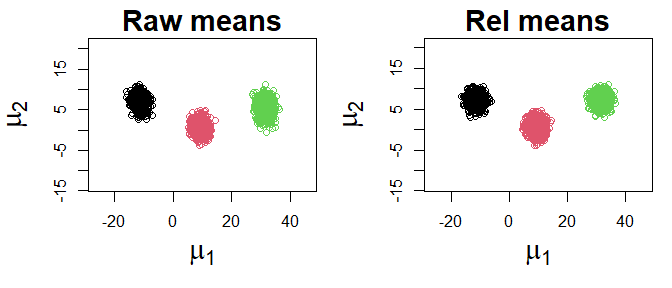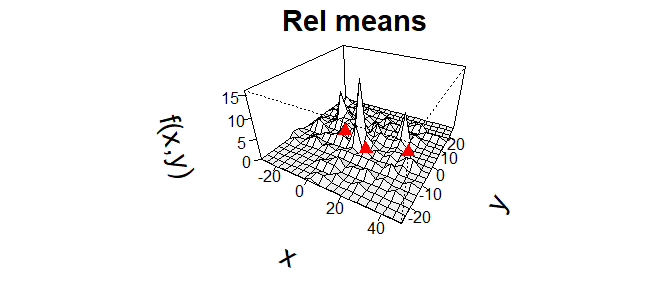Example: fishery data The Fishery dataset has been previously used by Titterington, Smith, and Makov (1985) and Papastamoulis (2016) and consists of 256 snapper length measurements. It is contained in the bayesmix package (Grün 2011). We may display the histogram of the data, along with an estimated kernel density. data(fish) y <- fish[,1] hist(y, breaks=40, prob = TRUE, cex.lab=1.6, main ="Fishery data", cex.main =1.7, col="navajowhite1", border="navajowhite1") lines(density(y), lty=1, lwd=3, col="blue")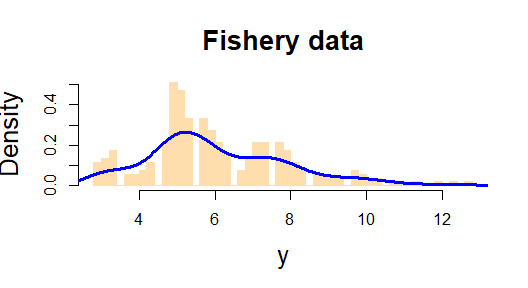We assume a mixture model with $$k=5$$ groups: $\begin{equation} y_i \sim \sum_{j=1}^k \eta_j \mathcal{N}(\mu_j, \sigma^2_j), \ \ i=1,\ldots,n, \label{eq:fishery} \end{equation}$ where $$\mu_j, \sigma_j$$ are the mean and the standard deviation of group $$j$$, respectively. Moreover, assume that $$z_1,\ldots,z_n$$ is an unobserved latent sequence of i.i.d. random variables following the multinomial distribution with weights $$\boldsymbol{\eta}=(\eta_{1},\dots,\eta_{k})$$, such that: $P(z_i=j)=\eta_j,$ where $$\eta_j$$ is the mixture weight assigned to the group $$j$$. We fit our model by simulating $$H=15000$$ samples from the posterior distribution of $$(\boldsymbol{z}, \boldsymbol{\mu}, \boldsymbol{\sigma}, \boldsymbol{\eta})$$. In the univariate case, if the argument software="rjags" is selected (the default option), Gibbs sampling is performed by the package bayesmix, and the priors are: $\begin{eqnarray} \mu_j \sim & \mathcal{N}(\mu_0, 1/B_0)\\ \sigma_j \sim & \mbox{invGamma}(\nu_0/2, \nu_0S_0/2)\\ \eta \sim & \mbox{Dirichlet}(\boldsymbol{\alpha})\\ S_0 \sim & \mbox{Gamma}(g_0/2, g_0G_0/2), \end{eqnarray}$ with default values: $$B_0=0.1$$, $$\nu_0 =20$$, $$g_0 = 10^{-16}$$, $$G_0 = 10^{-16}$$, $$\boldsymbol{\alpha}=(1,1,\ldots,1)$$. The users may specify their own hyperparameters with the priors arguments, declaring a names list such as: priors = list(mu_0=2, alpha = rep(2, k), ...). If software="rstan" is selected, the priors are: $\begin{eqnarray} \mu_j & \sim \mathcal{N}(\mu_0, 1/B0inv)\\ \sigma_j & \sim \mbox{Lognormal}(\mu_{\sigma}, \tau_{\sigma})\\ \eta_j & \sim \mbox{Uniform}(0,1), \end{eqnarray}$ where the vector of the weights $$\boldsymbol{\eta}=(\eta_1,\ldots,\eta_k)$$ is a $$k$$-simplex. Default hyperparameters values are: $$\mu_0=0, B0inv=0.1, \mu_{\sigma}=0, \tau_{\sigma}=2$$. Here also, the users may choose their own hyperparameters values in the following way: priors = list(mu_sigma = 0, tau_sigma = 1,...). We fit the model using the rjags method, and we set the burnin period to 7500. k <- 5 nMC <- 15000 res <- piv_MCMC(y = y, k = k, nMC = nMC, burn = 0.5*nMC, software = "rjags") #> Compiling model graph #> Declaring variables #> Resolving undeclared variables #> Allocating nodes #> Graph information: #> Observed stochastic nodes: 256 #> Unobserved stochastic nodes: 268 #> Total graph size: 1050 #> #> Initializing model #> #> #> Call: #> JAGSrun(y = y, model = mod.mist.univ, control = control) #> #> Markov Chain Monte Carlo (MCMC) output: #> Start = 7501 #> End = 22500 #> Thinning interval = 1 #> #> Empirical mean, standard deviation and 95% CI for eta #> Mean SD 2.5% 97.5% #> eta 0.2030 0.20230 0.009156 0.5672 #> eta 0.2006 0.13765 0.009236 0.5198 #> eta 0.1674 0.12353 0.018239 0.5051 #> eta 0.1217 0.05231 0.071350 0.1988 #> eta 0.3073 0.22254 0.009916 0.5752 #> #> Empirical mean, standard deviation and 95% CI for mu #> Mean SD 2.5% 97.5% #> mu 8.386 2.7806 3.377 12.341 #> mu 8.413 2.1134 5.178 12.347 #> mu 8.369 1.5851 5.201 10.862 #> mu 3.472 0.6172 3.126 5.368 #> mu 7.358 2.7863 5.069 12.292 #> #> Empirical mean, standard deviation and 95% CI for sigma2 #> Mean SD 2.5% 97.5% #> sigma2 0.4473 0.2510 0.1932 1.1808 #> sigma2 0.4387 0.2108 0.2009 1.0234 #> sigma2 0.4526 0.2415 0.2021 1.1223 #> sigma2 0.2606 0.1128 0.1280 0.5479 #> sigma2 0.4132 0.2442 0.2035 1.1182 First of all, we may access the true number of iterations by tiping: res$true.iter
#>  7421

We may have a glimpse if label switching ocurred or not by looking at the traceplot for the mean parameters, $$\mu_j$$. To do this, we apply the function piv_rel to relabel the chains and obtain useful inferences; the only argument for this function is the MCMC result just obtained with piv_MCMC. The function piv_plot displays some graphical tools, both traceplots (argument type="chains") and histograms along with the final relabelled means (argument type="hist"). For both plot ttpes, the function returns a printed message explaining how to interpret the results.

rel <- piv_rel(mcmc=res)
piv_plot(y=y, res, rel, par = "mean", type="chains")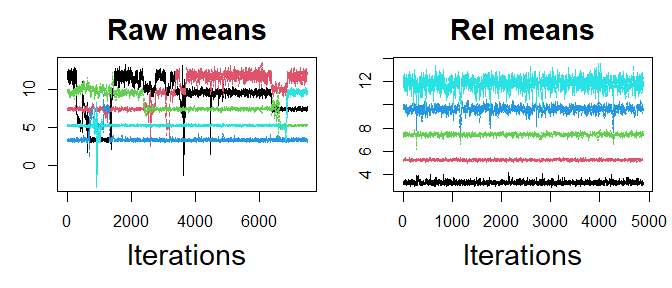#> Description: traceplot of the raw MCMC chains and the relabelled chains for the means parameters. Each colored chain corresponds to one of the k distinct parameters of the mixture model. Overlapping chains may reveal that the MCMC sample is not able to distinguish between the components.
piv_plot(y=y, res, rel, type="hist")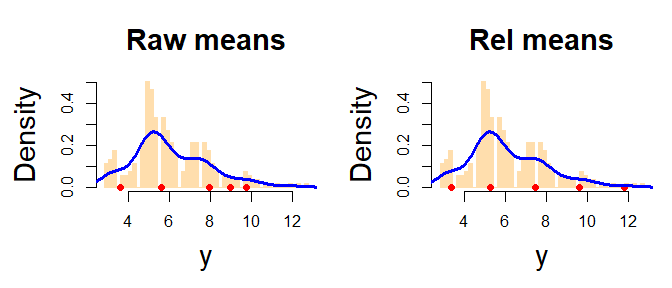#> Description: histograms of the data along with the estimated posterior means (red points) from raw MCMC and relabelling algorithm. The blue line is the estimated density curve.

The first plot displays the traceplots for the parameters $$\boldsymbol{\mu}$$. From the left plot showing the raw outputs as given by the Gibbs sampling, we note that label switching clearly occurred. Our algorithm seems able to reorder the mean $$\mu_j$$ and the weights $$\eta_j$$, for $$j=1,\ldots,k$$. Of course, a MCMC sampler which does not switch the labels would ideal, but nearly impossible to program. However, we could assess how two diferent sampler perform, by repeating the analysis above by selecting software="rstan" in the piv_MCMC function.

# stan code not evaluated here
res2 <- piv_MCMC(y = y, k = k, nMC = 3000,
software = "rstan")
rel2 <- piv_rel(res2)
piv_plot(y=y, res2, rel2, par = "mean", type="chains")

With the rstan option, we can use the bayesplot functions on the argument:

# stan code not evaluated here
posterior <- as.array(res2$stanfit) mcmc_intervals(posterior, regex_pars = c("mu")) Regardless of the software that we chose, we may extract the JAGS/Stan model by typing: cat(res$model)
#> var
#>  b0,
#>      B0inv,
#>      nu0Half,
#>      g0Half,
#>      g0G0Half,
#>      k,
#>      N,
#>      eta,
#>  mu,
#>  tau,
#>  nu0S0Half,
#>      S0,
#>      e,
#>  y,
#>  S;
#>
#> model    {
#>  for (i in 1:N) {
#>      y[i] ~ dnorm(mu[S[i]],tau[S[i]]);
#>      S[i] ~ dcat(eta[]);
#>  }
#>  for (j in 1:k) {
#>      mu[j] ~ dnorm(b0,B0inv);
#>      tau[j] ~ dgamma(nu0Half,nu0S0Half);
#>  }
#>  S0 ~ dgamma(g0Half,g0G0Half);
#>  nu0S0Half <- nu0Half * S0;
#>
#>  eta[] ~ ddirch(e[]);
#> }